# The temperature 14

The temperature is 12°C lower than 4 hours ago.

a. What is the average temperature change per hour?

b. Write an integer equation for the situation.

t =  3 °C/h

### Step-by-step explanation: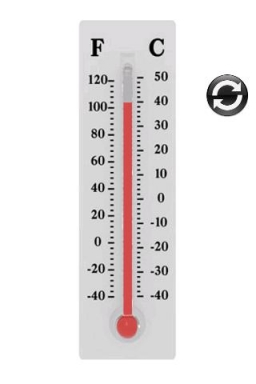Did you find an error or inaccuracy? Feel free to write us. Thank you!Tips to related online calculators
Looking for help with calculating arithmetic mean?
Looking for a statistical calculator?

#### You need to know the following knowledge to solve this word math problem:

We encourage you to watch this tutorial video on this math problem:

## Related math problems and questions:

• Change per hour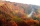On Sunday, the temperature was 63 degrees at 2 pm. The temperature was then 72 degrees at 5 pm. What is the rate of change per hour?
• Now isNow is 5°C morning. What will the temperature be if i. It rises by 12°C ii. It falls by 15°C
• The temperature 13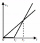The temperature in Toronto at noon during a winter day measured 4°C. The temperature started dropping 2° every hour. Which inequality can be used to find the number of hours, x, after which the temperature will measure below -3°C?
• RoxvilleThe temperature in Roxville decreased 15 degree in five hours. What was the average decrease in temperature per hour? Write you answer as an integer.
• WeatherWeather. At 5:00 am, the temperature was 28°C, and at noontime, it rose by 9°C. By 4:00 pm, it dropped 3°C, and by nighttime, it was 4°C less. What is the room temperature this time?
• ThereThere is 5 l of water with a temperature of 20°C in the bucket. We add 3 liters of hot water. The resulting temperature will be 30°C. What was the temperature of the hot water?
• Change in temperatureStarting temperature is 21°C, the highest temperature is 32°C. What is the change in temperature?
• Temperature 13Work out the temperature after each change. The temperature starts at six °C and falls by 13°C. The temperature starts at two °C and falls by eight °C
• Water temperaturesTo 25 liters of water at 50 °C we add 15 liters of water at a different temperature. How many °C should the water be colder than 50 °C so that 40 liters of the water obtained has a temperature 42.5 °C?
• The temperature 16The temperature at 6 am was 21 degrees Fahrenheit by noon, and the temperature dropped 32 degrees Fahrenheit. What was the temperature at noon?
• The bathDad poured 50 liters of water at 60°C into the tub. How much must cold water be added at 10°C to obtain a bath that has a temperature of 40°C?
• Water mixingWe have 520 ml of hot water and 640 ml of water at 48°C. What is the temperature of approximately hot water when the resulting mixture has a temperature of 65°C?
• Outdoor temperatureThe outdoor temperature was 8 degrees at midnight. The temperature declined 5 degrees during each of the next 3 hours. What was the temperature at 3am?
• Freezer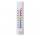The temperature is -8 degrees Celsius in the freezer. The outside temperature is 2 degrees Celsius. One minute took to change the temperature by one degree Celsius if we open the door. How many minutes the temperature in the freezer will rise?
• Temperature differenceLibya's highest temperature was recorded at 58 degrees Celsius, and the lowest was recorded at -88 degrees Celsius. What is the temperature difference?
• Daily average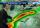Calculate the average temperature during the day, when 15 hours were 20 °C and 9 hours was 13 °C.
• Temperature changeThe mean temperature change is -3.2°F per day for five days. What is the total change over the five day period?